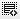## QUIZ Question of L440
If you took \$20 million as debt to fund a \$200 million project. Assuming 10% interest on the debt and returns of \$500 million in 5 years. What is your ROI?0

n this question Debt is 20 million and interest 10% so after 5 year you have to return 30 million....if expected return after 5 years is 500 million then left over is 470 million and your investment is 180 million so ROI = 470/180=261%0

Sir, but according to the question, the options given for the answer are incorrect. The answer calculated by taking the values given in the question does not match any of the answers given in the options.....0

Yes, the correct answer is not there in the options.

Click on thisicon to add code snippet.